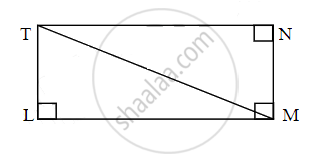# Find the diagonal of a rectangle whose length is 16 cm and area is 192 sq.cm. Complete the following activity. Activity: As shown in figure LMNT is reactangle.∴ Area of rectangle = length × breadth - Geometry

Sum

Find the diagonal of a rectangle whose length is 16 cm and area is 192 sq.cm. Complete the following activity.Activity: As shown in figure LMNT is a reactangle.
∴ Area of rectangle = length × breadth

∴ Area of rectangle = square × breadth

∴ 192 = square × breadth

Also,

∠TLM = 90°    ......[Each angle of reactangle is right angle]

In ∆TLM,

By Pythagoras theorem

∴ TM2 = TL2 + square

∴ TM2 = 122 + square

∴ TM2 = 144 + square

∴ TM2 = 400

∴ TM = 20

#### Solution

As shown in figure LMNT is reactangle.

∴ Area of rectangle = length × breadth

∴ Area of rectangle = 16 × breadth

∴ 192 = 16 × breadth

∴ Breadth = 192/16

i.e., TL = 12 cm and LM = 16 cm   ......(i)

Also, ∠TLM = 90°    ......[Each angle of reactangle is right angle]

In ∆TLM, By Pythagoras theorem

∴ TM2 = TL2 + LM2

∴ TM2 = 122 + 162     ......[From (i)]

∴ TM2 = 144 + 256

∴ TM2 = 400

∴ TM = 20

Concept: Similarity in Right Angled Triangles
Is there an error in this question or solution?

Share